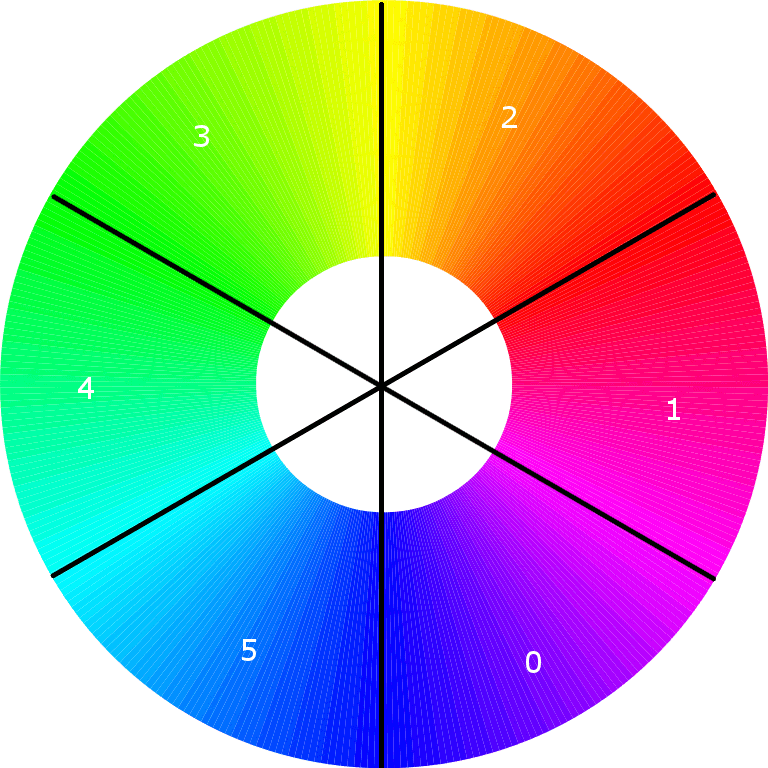# RGB Rainbow

Apr 5, 2018 • Arne Vogel

For the cyclic cellular automaton I had to get a variable amount of colors around the color circle. This is how I solved that problem.

\# of colors
I split the color wheel into 6 equal parts:Sector/Color R G B
0 incr. 0 255
1 255 0 decr.
2 255 incr. 0
3 decr. 255 0
4 0 255 incr.
5 0 decr. 255

incr. means the color is increasing and decr. means the color is decreasing going counter clockwise around the circle.

The pseudo code to achieve this:

``````#get the i'th color, of n colors.
color(i,n):
r, g, b = 0
stepsize = floor((255*6)/n)
progress = i*stepsize
switch(floor(progress/255))
case 0:
b = 255
r = progress%255
case 1:
r = 255
b = progress%255
case 2:
r = 255
g = progress%255
case 3:
g = 255
r = progress%255
case 4:
g = 255
b = progress%255
case 5:
b = 255
g = progress%255
return r, g, b
``````
\# of colors
## Attributions RGB color wheel: https://commons.wikimedia.org/wiki/File:RGB_color_wheel_360.svg